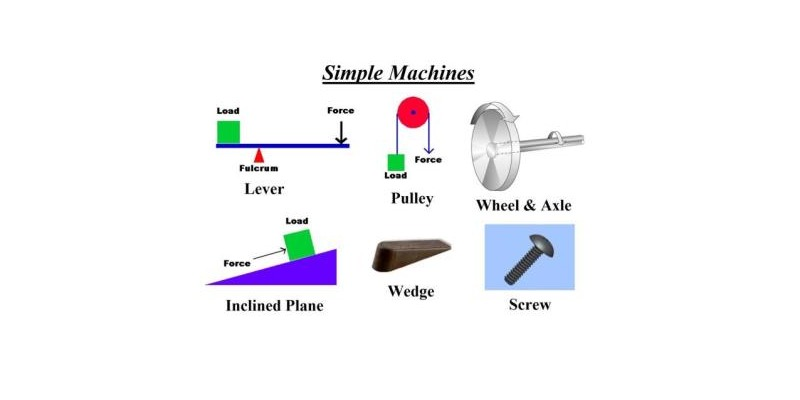### Ultimate Physics Trivia Quiz For 6th Grade StudentsPhysics is a branch of science and the main goal of this subject is to understand how the universe behaves. If you are in sixth grade and science is your favorite subject this test is for you. In this quiz, you can learn physics basics knowledge. So let's start this quiz

#### 1. The SI unit of speed is ...

 81101 m/s 81102 Km/h 81103 c/m 81104 s/m

#### 2. The SI unit of force ...

 81105 Watt 81106 Newton 81107 Kilogram 81108 Kilometer

#### 3. What is the symbol of mass?

 81109 m 81110 M 81111 Both of these 81112 None of these

#### 4. What is the symbol of wavelength?

 81113 θ 81114 ω 81115 λ 81116 T

#### 5. What is the symbol of resistance?

 81117 R 81118 M 81119 C 81120 H

#### 6. Product of force and velocity is called...

 81121 Motion 81122 Power 81123 Work 81124 Energy

#### 7. Which law is also called the law of inertia?

 81125 Motion first law 81126 Gravitational law 81127 Newton's first law 81128 None of these

#### 8. What is the unit of astronomical distance?

 81129 Newton 81130 Sun year 81131 Light year 81132 None of these

#### 9. What is the scale used for measuring the intensity of the earthquake ?

 81133 Meter scale 81134 Richter Scale 81135 Light Scale 81136 All of above

#### 10. When was newton born?

 81137 4 January 1643 81138 4 January 1743 81139 26 January 1643 81140 14 January 1645